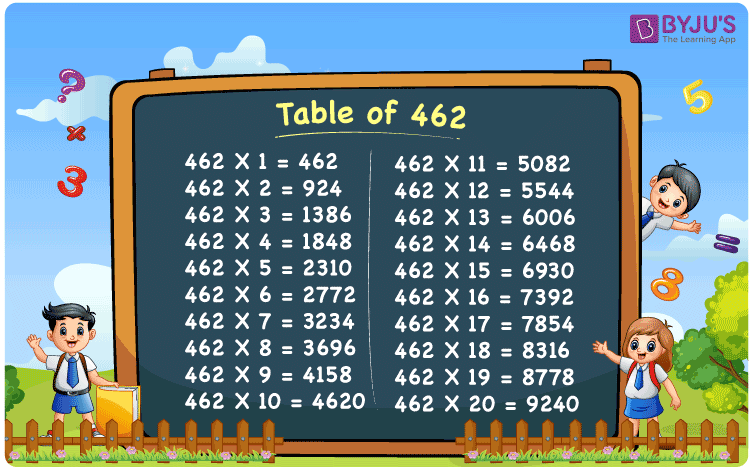Checkout JEE MAINS 2022 Question Paper Analysis : Checkout JEE MAINS 2022 Question Paper Analysis :

# Table of 462

The 462 Multiplication Table is nothing more than the addition of the prime number 462 over and over again. Since the digits at these locations are multiplied by 1, the easiest method to memorise the table of 462 is to look for repetitions of the number at the one’s and hundred’s places. You can use the Multiplication calculator for any multiplication table, if you need further help.

## Table of 462 Chart## What is the 462 Times Table?

A multiplication table is nothing but a repetitive addition. In this table we are repeatedly adding 462. In a tabular format, the repetitive addition looks like this.

 462×1 = 462 462 462×2 = 924 462 + 462 = 924 462×3 = 1386 462 + 462 + 462 = 1386 462×4 = 1848 462 + 462 + 462 + 462 = 1848 462×5 = 2310 462 + 462 + 462 + 462 + 462 = 2310 462×6 = 2772 462 + 462 + 462 + 462 + 462 + 462 = 2772 462×7 = 3234 462 + 462 + 462 + 462 + 462 + 462 + 462 = 3234 462×8 = 3696 462 + 462 + 462 + 462 + 462 + 462 + 462 + 462 = 3696 462×9 = 4158 462 + 462 + 462 + 462 + 462 + 462 + 462 + 462 + 462 = 4158 462×10 = 4620 462 + 462 + 462 + 462 + 462 + 462 + 462 + 462 + 462 + 462 = 4620

## Multiplication Table of 462

The multiplication table of 462 is given below in tabular form:

 462 × 1 = 462 462 × 2 = 924 462 × 3 = 1386 462 × 4 = 1848 462 × 5 = 2310 462 × 6 = 2772 462 × 7 = 3234 462 × 8 = 3696 462 × 9 = 4158 462 × 10 = 4620 462 × 11 = 5082 462 × 12 = 5544 462 × 13 = 6006 462 × 14 = 6468 462 × 15 = 6930 462 × 16 = 7392 462 × 17 = 7854 462 × 18 = 8316 462 × 19 = 8778 462 × 20 = 9240

## Solved Example on Table of 462

Q. What is the sum of the 14th multiple and the 15th multiple of 462?

Solution:

Using the multiplication table of 462

The 14th multiple of 462 = 6468

The 15th multiple of 462 = 6930

The difference between the 14th multiple and 15th multiple = 6468 + 6930 = 13398

## Frequently Asked Questions on the Table of 462

### What is the table of 462?

The table of 462 is the list of all multiples of 462 starting from 462 × 1 = 462, 462 × 2 = 924, 462 × 3 = 1386, and so on.

### What is 19 times 462?

19 times 462 is 8778.

### Calculate the value 462 times 10 minus 20.

462 times 10 minus 20 equals 4600.
I.e., 462 × 10 – 20 = 4600.